# e constant

e constant or Euler's number is a mathematical constant. The e constant is real and irrational number.

e = 2.718281828459...

## Definition of e

The e constant is defined as the limit: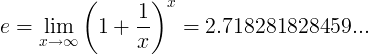#### Alternative definitions

The e constant is defined as the limit: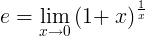The e constant is defined as the infinite series: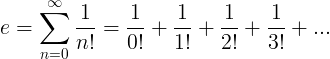## Properties of e

#### Reciprocal of e

The reciprocal of e is the limit: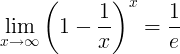#### Derivatives of e

The derivative of the exponential function is the exponential function:

(e x)' = ex

The derivative of the natural logarithm function is the reciprocal function:

(loge x)' = (ln x)' = 1/x

#### Integrals of e

The indefinite integral of the exponential function ex is the exponential function ex.

ex dx = ex+c

The indefinite integral of the natural logarithm function loge x is:

∫ loge x dx = ∫ lnx dx = x ln x - x +c

The definite integral from 1 to e of the reciprocal function 1/x is 1: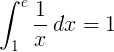## Base e logarithm

The natural logarithm of a number x is defined as the base e logarithm of x:

ln x = loge x

## Exponential function

The exponential function is defined as:

f (x) = exp(x) = ex

## Euler's formula

The complex number e has the identity:

e = cos(θ) + i sin(θ)

i is the imaginary unit (the square root of -1).

θ is any real number.

### How to find a number in e?

Finding a number in e digits necessitates to browse all its decimals, there is no other mean. There are no mean to predict a number and its position in $e$.

Example: 8 is is position 3 and 5 (and many others)

For decoding the first decimal 7 in 2.71828 is in position 1. Some programs take the same position as 0 (some computers count from 0), others consider the position 2 (counting the number 2 before the decimal dot/point as the position 1).

### What are the names for e?

e is also called Euler's number or Napier's constant.

### How many digits are there in e decimals?

There are an infinite number of decimal places in the number e=2.71828... Supercomputers are continuing to find more. dCode knows the e decimals until 1000000 (one million digits of e).

Currently, we have around 5610 calculators, conversion tables and usefull online tools and software features for students, teaching and teachers, designers and simply for everyone.